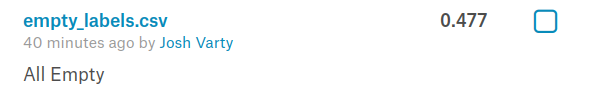# Probing Kaggle Leaderboards with Dice Score

This post was based on the discussion posted by Bibek here and the related discussion posted by Heng CherKeng here.

In the Understanding Clouds from Satellite Images Kaggle contest, competitors are challenged to identify and segment different cloud formations in a series of satellite photos. There are four different kinds of cloud formations we are tasked with identifying. The lines between them can be fuzzy at times, but they’re described as follows:Given a satellite photo, we’re challenged to identify these formations and produce an output similar to:In order to compare submissions against one another, the competition organizers decided to use the metric Dice Score. It’s formula is given as:Where:

• X is the set of our predictions
• Y is the set of ground truth labels (1 for True, 0 for False)

One important note is that Dice Score is defined as 1 whenever X and Y are empty. This leads to an interesting property that will allow us to probe the leaderboard for useful information.

Before we do that, let’s look at a few examples on super small 4x4 images:

Predictions (X)Labels (Y)$Dice Score = \frac{2*{(0.50 * 1.00 +0.75*1.00+0.01*0.00+0.01*0.00)}}{(0.50 + 0.75+0.01+0.01) + (1.00+1.00+0.00+0.00)} = 0.76$

For an image with no labels where we predict very small probabilities:

Predictions (X)Labels (Y)$Dice Score = \frac{2*{(0.01 * 0.00 +0.00*0.00+0.00*0.00+0.00*0.00)}}{(0.01+0.00+0.00+0.00) + (0.00+0.00+0.00+0.00)} = 0.00$

That weird… Even though we’re very, very close to the right answer we get the worst possible Dice Score. If we had left that first position empty, we would have had a perfect Dice Score (since Dice Score is defined as 1 whenever both sets are empty).

This property allows us to probe the Kaggle leaderboard for some interesting information.

In our contest there are 3,698 test images. Since there are four possible classes (Sugar, Flower, Gravel and Fish) we need to make four sets of predictions (or masks) for each image. This means we’ll be making 14,792 sets of predictions in total.

If we submit a set of empty predictions, we can use our score to calculate how many empty masks are present in the total set*.So an empty submission gets us a score of 0.477. We know that each time we made a correct empty prediction we got a perfect Dice Score of 1.0, and we know that each time we made an incorrect empty prediction we got the worst Dice Score of 0.0. This means we can calculate the total number of empty masks.

0.477 * 14,792 = ~7,055 empty masks.

We can go further and calculate the exact number of empty masks for each class.

Let’s take take our empty predictions for a single class and replace them with a bad prediction (eg. a single pixel in the top-left corner). On every single empty mask for this class, our score will drop from 1.0 to 0.0.** We can use this drop to calculate how many empty masks must have been present.

When we alter our predictions for Sugar our score drops to:When we alter our predictions for Gravel our score drops to:When we alter our predictions for Flower our score drops to:When we alter our predictions for Fish our score drops to:Given the change in score, we can calculate the empty labels for each class as follows:

Sugar

(0.477 – 0.388) * 14,792 = ~1316 empty masks for Sugar

Gravel

(0.477 – 0.361) * 14,792 = ~1716 empty masks for Gravel

Flower

(0.477 – 0.329) * 14,792 = ~2190 empty masks for Flower

Fish

(0.477 – 0.353) * 14,792 = ~1834 empty Masks for Fish

So what can we do with this information? The most obvious strategy would be to use this information when creating a validation set. Normally this would help us achieve a more accurate validation set (Why Validation Sets Matter) but there’s something strange going on with this particular competition that suggests a stronger validation set might not actually help.

An alternate use for this technique would be to help in verifying a data leak. I suspect that such a leak exists and that one can get a perfect score on a portion of the images in the test set. We could use this technique to verify the leak by modifying an empty submission with a handful of “perfect” submissions and observing if our score increases by the predicted amount.

* In reality, the resulting score we’re looking at is calculated on only 25% of the data. After the contest concludes, the organizers will re-run our submissions on the full dataset. It’s worth being aware of the possibility that the 25% of the data we’re being graded on may have been specifically chosen to be misleading, but for the remainder of this post we’ll assume it’s representative of the entire test set.

** Technically we might accidentally hit a correct label in some of the non-empty masks, but the change to our Dice Score will be so small that we can ignore this case.